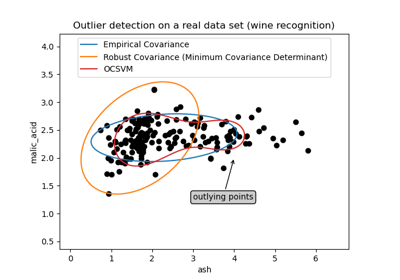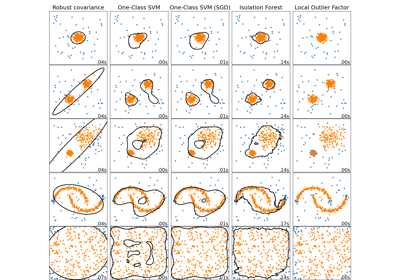# sklearn.covariance.EllipticEnvelope¶

class sklearn.covariance.EllipticEnvelope(*, store_precision=True, assume_centered=False, support_fraction=None, contamination=0.1, random_state=None)[source]

An object for detecting outliers in a Gaussian distributed dataset.

Read more in the User Guide.

Parameters:
store_precisionbool, default=True

Specify if the estimated precision is stored.

assume_centeredbool, default=False

If True, the support of robust location and covariance estimates is computed, and a covariance estimate is recomputed from it, without centering the data. Useful to work with data whose mean is significantly equal to zero but is not exactly zero. If False, the robust location and covariance are directly computed with the FastMCD algorithm without additional treatment.

support_fractionfloat, default=None

The proportion of points to be included in the support of the raw MCD estimate. If None, the minimum value of support_fraction will be used within the algorithm: [n_sample + n_features + 1] / 2. Range is (0, 1).

contaminationfloat, default=0.1

The amount of contamination of the data set, i.e. the proportion of outliers in the data set. Range is (0, 0.5].

random_stateint, RandomState instance or None, default=None

Determines the pseudo random number generator for shuffling the data. Pass an int for reproducible results across multiple function calls. See Glossary.

Attributes:
location_ndarray of shape (n_features,)

Estimated robust location.

covariance_ndarray of shape (n_features, n_features)

Estimated robust covariance matrix.

precision_ndarray of shape (n_features, n_features)

Estimated pseudo inverse matrix. (stored only if store_precision is True)

support_ndarray of shape (n_samples,)

A mask of the observations that have been used to compute the robust estimates of location and shape.

offset_float

Offset used to define the decision function from the raw scores. We have the relation: decision_function = score_samples - offset_. The offset depends on the contamination parameter and is defined in such a way we obtain the expected number of outliers (samples with decision function < 0) in training.

New in version 0.20.

raw_location_ndarray of shape (n_features,)

The raw robust estimated location before correction and re-weighting.

raw_covariance_ndarray of shape (n_features, n_features)

The raw robust estimated covariance before correction and re-weighting.

raw_support_ndarray of shape (n_samples,)

A mask of the observations that have been used to compute the raw robust estimates of location and shape, before correction and re-weighting.

dist_ndarray of shape (n_samples,)

Mahalanobis distances of the training set (on which fit is called) observations.

n_features_in_int

Number of features seen during fit.

New in version 0.24.

feature_names_in_ndarray of shape (n_features_in_,)

Names of features seen during fit. Defined only when X has feature names that are all strings.

New in version 1.0.

EmpiricalCovariance

Maximum likelihood covariance estimator.

GraphicalLasso

Sparse inverse covariance estimation with an l1-penalized estimator.

LedoitWolf

LedoitWolf Estimator.

MinCovDet

Minimum Covariance Determinant (robust estimator of covariance).

OAS

Oracle Approximating Shrinkage Estimator.

ShrunkCovariance

Covariance estimator with shrinkage.

Notes

Outlier detection from covariance estimation may break or not perform well in high-dimensional settings. In particular, one will always take care to work with n_samples > n_features ** 2.

References



Rousseeuw, P.J., Van Driessen, K. “A fast algorithm for the minimum covariance determinant estimator” Technometrics 41(3), 212 (1999)

Examples

>>> import numpy as np
>>> from sklearn.covariance import EllipticEnvelope
>>> true_cov = np.array([[.8, .3],
...                      [.3, .4]])
>>> X = np.random.RandomState(0).multivariate_normal(mean=[0, 0],
...                                                  cov=true_cov,
...                                                  size=500)
>>> cov = EllipticEnvelope(random_state=0).fit(X)
>>> # predict returns 1 for an inlier and -1 for an outlier
>>> cov.predict([[0, 0],
...              [3, 3]])
array([ 1, -1])
>>> cov.covariance_
array([[0.7411..., 0.2535...],
[0.2535..., 0.3053...]])
>>> cov.location_
array([0.0813... , 0.0427...])


Methods

 Apply a correction to raw Minimum Covariance Determinant estimates. Compute the decision function of the given observations. error_norm(comp_cov[, norm, scaling, squared]) Compute the Mean Squared Error between two covariance estimators. fit(X[, y]) Fit the EllipticEnvelope model. fit_predict(X[, y]) Perform fit on X and returns labels for X. Get metadata routing of this object. get_params([deep]) Get parameters for this estimator. Getter for the precision matrix. Compute the squared Mahalanobis distances of given observations. Predict labels (1 inlier, -1 outlier) of X according to fitted model. Re-weight raw Minimum Covariance Determinant estimates. score(X, y[, sample_weight]) Return the mean accuracy on the given test data and labels. Compute the negative Mahalanobis distances. set_params(**params) Set the parameters of this estimator. set_score_request(*[, sample_weight]) Request metadata passed to the score method.
correct_covariance(data)[source]

Apply a correction to raw Minimum Covariance Determinant estimates.

Correction using the empirical correction factor suggested by Rousseeuw and Van Driessen in [RVD].

Parameters:
dataarray-like of shape (n_samples, n_features)

The data matrix, with p features and n samples. The data set must be the one which was used to compute the raw estimates.

Returns:
covariance_correctedndarray of shape (n_features, n_features)

Corrected robust covariance estimate.

References

[RVD]

A Fast Algorithm for the Minimum Covariance Determinant Estimator, 1999, American Statistical Association and the American Society for Quality, TECHNOMETRICS

decision_function(X)[source]

Compute the decision function of the given observations.

Parameters:
Xarray-like of shape (n_samples, n_features)

The data matrix.

Returns:
decisionndarray of shape (n_samples,)

Decision function of the samples. It is equal to the shifted Mahalanobis distances. The threshold for being an outlier is 0, which ensures a compatibility with other outlier detection algorithms.

error_norm(comp_cov, norm='frobenius', scaling=True, squared=True)[source]

Compute the Mean Squared Error between two covariance estimators.

Parameters:
comp_covarray-like of shape (n_features, n_features)

The covariance to compare with.

norm{“frobenius”, “spectral”}, default=”frobenius”

The type of norm used to compute the error. Available error types: - ‘frobenius’ (default): sqrt(tr(A^t.A)) - ‘spectral’: sqrt(max(eigenvalues(A^t.A)) where A is the error (comp_cov - self.covariance_).

scalingbool, default=True

If True (default), the squared error norm is divided by n_features. If False, the squared error norm is not rescaled.

squaredbool, default=True

Whether to compute the squared error norm or the error norm. If True (default), the squared error norm is returned. If False, the error norm is returned.

Returns:
resultfloat

The Mean Squared Error (in the sense of the Frobenius norm) between self and comp_cov covariance estimators.

fit(X, y=None)[source]

Fit the EllipticEnvelope model.

Parameters:
Xarray-like of shape (n_samples, n_features)

Training data.

yIgnored

Not used, present for API consistency by convention.

Returns:
selfobject

Returns the instance itself.

fit_predict(X, y=None, **kwargs)[source]

Perform fit on X and returns labels for X.

Returns -1 for outliers and 1 for inliers.

Parameters:
X{array-like, sparse matrix} of shape (n_samples, n_features)

The input samples.

yIgnored

Not used, present for API consistency by convention.

**kwargsdict

Arguments to be passed to fit.

New in version 1.4.

Returns:
yndarray of shape (n_samples,)

1 for inliers, -1 for outliers.

Get metadata routing of this object.

Please check User Guide on how the routing mechanism works.

Returns:

A MetadataRequest encapsulating routing information.

get_params(deep=True)[source]

Get parameters for this estimator.

Parameters:
deepbool, default=True

If True, will return the parameters for this estimator and contained subobjects that are estimators.

Returns:
paramsdict

Parameter names mapped to their values.

get_precision()[source]

Getter for the precision matrix.

Returns:
precision_array-like of shape (n_features, n_features)

The precision matrix associated to the current covariance object.

mahalanobis(X)[source]

Compute the squared Mahalanobis distances of given observations.

Parameters:
Xarray-like of shape (n_samples, n_features)

The observations, the Mahalanobis distances of the which we compute. Observations are assumed to be drawn from the same distribution than the data used in fit.

Returns:
distndarray of shape (n_samples,)

Squared Mahalanobis distances of the observations.

predict(X)[source]

Predict labels (1 inlier, -1 outlier) of X according to fitted model.

Parameters:
Xarray-like of shape (n_samples, n_features)

The data matrix.

Returns:
is_inlierndarray of shape (n_samples,)

Returns -1 for anomalies/outliers and +1 for inliers.

reweight_covariance(data)[source]

Re-weight raw Minimum Covariance Determinant estimates.

Re-weight observations using Rousseeuw’s method (equivalent to deleting outlying observations from the data set before computing location and covariance estimates) described in [RVDriessen].

Parameters:
dataarray-like of shape (n_samples, n_features)

The data matrix, with p features and n samples. The data set must be the one which was used to compute the raw estimates.

Returns:
location_reweightedndarray of shape (n_features,)

Re-weighted robust location estimate.

covariance_reweightedndarray of shape (n_features, n_features)

Re-weighted robust covariance estimate.

support_reweightedndarray of shape (n_samples,), dtype=bool

A mask of the observations that have been used to compute the re-weighted robust location and covariance estimates.

References

A Fast Algorithm for the Minimum Covariance Determinant Estimator, 1999, American Statistical Association and the American Society for Quality, TECHNOMETRICS

score(X, y, sample_weight=None)[source]

Return the mean accuracy on the given test data and labels.

In multi-label classification, this is the subset accuracy which is a harsh metric since you require for each sample that each label set be correctly predicted.

Parameters:
Xarray-like of shape (n_samples, n_features)

Test samples.

yarray-like of shape (n_samples,) or (n_samples, n_outputs)

True labels for X.

sample_weightarray-like of shape (n_samples,), default=None

Sample weights.

Returns:
scorefloat

Mean accuracy of self.predict(X) w.r.t. y.

score_samples(X)[source]

Compute the negative Mahalanobis distances.

Parameters:
Xarray-like of shape (n_samples, n_features)

The data matrix.

Returns:
negative_mahal_distancesarray-like of shape (n_samples,)

Opposite of the Mahalanobis distances.

set_params(**params)[source]

Set the parameters of this estimator.

The method works on simple estimators as well as on nested objects (such as Pipeline). The latter have parameters of the form <component>__<parameter> so that it’s possible to update each component of a nested object.

Parameters:
**paramsdict

Estimator parameters.

Returns:
selfestimator instance

Estimator instance.

set_score_request(*, sample_weight: Union[bool, None, str] = '$UNCHANGED$') [source]

Request metadata passed to the score method.

Note that this method is only relevant if enable_metadata_routing=True (see sklearn.set_config). Please see User Guide on how the routing mechanism works.

The options for each parameter are:

• True: metadata is requested, and passed to score if provided. The request is ignored if metadata is not provided.

• False: metadata is not requested and the meta-estimator will not pass it to score.

• None: metadata is not requested, and the meta-estimator will raise an error if the user provides it.

• str: metadata should be passed to the meta-estimator with this given alias instead of the original name.

The default (sklearn.utils.metadata_routing.UNCHANGED) retains the existing request. This allows you to change the request for some parameters and not others.

New in version 1.3.

Note

This method is only relevant if this estimator is used as a sub-estimator of a meta-estimator, e.g. used inside a Pipeline. Otherwise it has no effect.

Parameters:
sample_weightstr, True, False, or None, default=sklearn.utils.metadata_routing.UNCHANGED

Metadata routing for sample_weight parameter in score.

Returns:
selfobject

The updated object.

## Examples using sklearn.covariance.EllipticEnvelope¶Outlier detection on a real data set

Outlier detection on a real data setComparing anomaly detection algorithms for outlier detection on toy datasets

Comparing anomaly detection algorithms for outlier detection on toy datasets